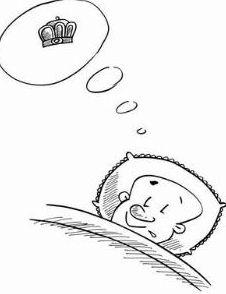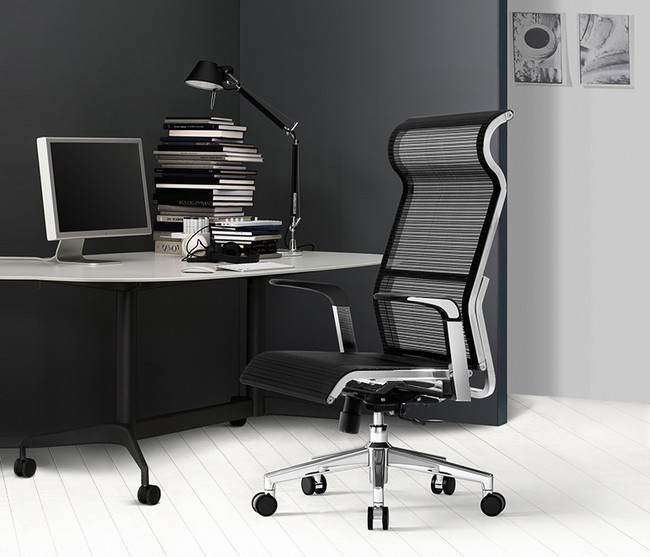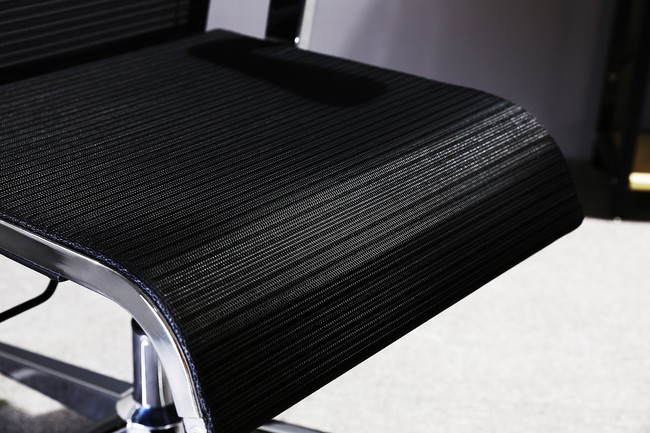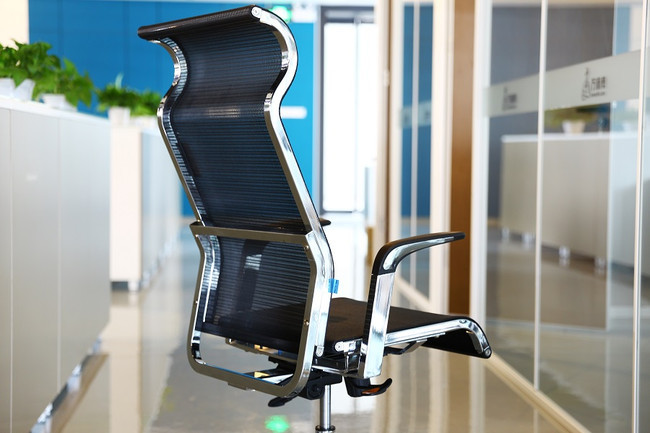|

# 测评:当办公遇上西昊 X1电脑椅欲发洪荒之力

从小就被教导一定要好好念书，将来成为社会栋梁，为国家和社会做出贡献，这种宏远的目标着实让人感觉是在“做白做梦”。可是，原来“好好念书”还是有好处的，比如它可以指引我们进入一家不错的企业。任何一家优质的企业是非常“善待”员工的，那些办公用具都是“往好里使”，比如办公桌椅的选购，一般的企业会因为考虑经费问题而选择一款价格、外观都还不错的产品。

作为一家有前瞻性的企业，往往会选择一款实用且具有人体工学理念的优质电脑椅，比如西昊品牌的核心产品——X1人体工学电脑椅，毕竟一款融入了科学概念的电脑椅可以为员工的健康带来福利，进而提高工作效率，为自己企业的未来发展助力，这就是“有心机”的企业。小编发现，现在已经有不少企业都开始注重员工们的“坐”文化，即使是个人，也对座椅的选择有了更高要求。毕竟一天中有八小时得“坐”在办公室，一天24小时的三分之一就这么耗上了，如果不选择一款好的座椅，对得住呕心沥血付出青春拼命工作的自己么?

小编不(废)遗(话)余(真)力(多)来一个较长的铺垫，但其实今天的最终目的是为给西昊X1人体工学电脑椅做一个测评，有兴趣的小伙伴最后别忘点个赞!

西昊X1电脑椅外观：品质出众 彰显时尚

星力指数：★★★★★

这个看脸的时代，哪都需要颜值在线!虽然西昊X1的颜值并不是所谓的“第一眼美女”，但绝对是“耐看型美女”。颜色主要是黑银两色，其实，黑银两色的拼接历来就是美学经典中的经典，永不落实，且始终名列时尚前茅，一件钟情。西昊X1不求花里胡哨、不要粉嫩可爱、不要古典高冷，但是却是沉稳和低调、内涵又奢华。毕竟这是在办公的地方使用，需要简约，但又不失大方。

另外从整个椅身来看，绝不呆板和笨重，有些细节之处还采用流线型，似乎让椅子看起来更性感。

西昊X1人体工学电脑椅网布：优质选材 透气舒适

星力指数：★★★★★

一款优质的椅子的用料是很讲究的。对于西昊X1电脑椅的网布选择，小编不从专业的角度为消费者进行分析，只是从直观的意义上来看，用手触摸后的网布十分顺滑，而且非常冰凉，比一般的电脑椅网布要低1-2度左右，尤其适合这种炎炎夏日使用。也就不会让我们的PP感到闷热啦!当坐下来的时候，弹性网面对于身体有一个很好的缓冲作用，但是又没有一种生硬的弹簧感，似乎是椅子给身体铸造的一个有安全感的柔软堡垒，这个体验舒适度感觉很不错。

西昊X1人体工学电脑椅腰托：加强护腰 线型腰托

星力指数：★★★★☆

西昊X1的背部有一个S型设计，这是一个护腰的细节处理。根据人体工学的特点，当我们坐下来的时候，腰部若与背部获得了最贴合的支撑，可以减轻腰部的支撑力，即使是久坐后也不会出现酸痛问题。小编坐上去对这个腰托进行体验时，分别做了上半身直立和后仰的实验，在腰背部直立时，背部仍能感受到最密切的贴合感;在后仰并做到最大化角度时，背部仍能获得有效支撑。

西昊X1人体工学电脑椅安全：防爆底盘 气压棒通过国际SGS认证

星力指数：★★★★★

现在对于电脑椅的选择，“安全性”也是我们必须考虑的因素。西昊作为人体工学电脑椅领导者，在安全性上倒也是做足了功夫。

小编从椅子的底部看到，这里有一个3毫米的加厚机构，可以让电脑椅更加稳固，给安全平添一个保障。小伙伴们无需担心这款椅子在长时间使用后会出现爆破的现象。

此外，电脑椅气压棒通过国际SGS认证，达到美国BFM标准，从源头保证椅子的安全!

西昊X1人体工学电脑椅制作：精工之选 钢铁之躯

星力指数：★★★★★

“千锤百炼出臻品”，小编实在找不到恰当的词句来形容这款电脑椅了。小编掂量了这款椅子，确实还是有点分量的。

每一个钢筋打磨的十分平滑，拼装起来就成了一个“不入之躯”，似乎无论怎样“打压”它，它都是顽强而有毅力，就算是对于一些“大老粗”，不怎么爱惜东西的小伙伴，想要把它玩坏，估计也得要费点时日才行。据说西昊X1的制作工序有55道，包括机床成型、手工打磨、数字铰孔、数控切割等等，着实把这款电脑椅打造成了“钢铁之躯”。

西昊X1人体工学电脑椅自由：灵活办公 职场任性

星力指数：★★★★★

“不会活动的椅子绝壁是一个假椅子，”有不少网友曾这样调侃过。西昊X1电脑椅也和其他的电脑椅一样，可以前、后、上、下任意调节。西昊X1可以360度旋转和10厘米上下自由活动，以及120度靠背自由锁定和8度坐垫联动后仰，时刻根据自己的需要打造最舒适的状态。西昊X1所设计的这些倾仰、旋转功能极富人性化概念，完全是由座椅来配合人体的倾仰动作，带给我们同步的健康与舒适。当坐上去之时，向后仰，椅座会有小幅度的上扬，这时只需要通过操纵杆调节坐深就能够调节，后仰时，双脚不会向上翘起，也不会出现大腿肌肉紧绷的状态，时刻保持着舒适模式。

`声明：本文由入驻焦点开放平台的作者撰写，除焦点官方账号外，观点仅代表作者本人，不代表焦点立场错误信息举报电话： 400-099-0099，邮箱：jubao@vip.sohu.com，或点此进行意见反馈，或点此进行举报投诉。`A B C D E F G H J K L M N P Q R S T W X Y Z
A - B - C - D - E
• A
• 鞍山
• 安庆
• 安阳
• 安顺
• 安康
• 澳门
• B
• 北京
• 保定
• 包头
• 巴彦淖尔
• 本溪
• 蚌埠
• 亳州
• 滨州
• 北海
• 百色
• 巴中
• 毕节
• 保山
• 宝鸡
• 白银
• 巴州
• C
• 承德
• 沧州
• 长治
• 赤峰
• 朝阳
• 长春
• 常州
• 滁州
• 池州
• 长沙
• 常德
• 郴州
• 潮州
• 崇左
• 重庆
• 成都
• 楚雄
• 昌都
• 慈溪
• 常熟
• D
• 大同
• 大连
• 丹东
• 大庆
• 东营
• 德州
• 东莞
• 德阳
• 达州
• 大理
• 德宏
• 定西
• 儋州
• 东平
• E
• 鄂尔多斯
• 鄂州
• 恩施
F - G - H - I - J
• F
• 抚顺
• 阜新
• 阜阳
• 福州
• 抚州
• 佛山
• 防城港
• G
• 赣州
• 广州
• 桂林
• 贵港
• 广元
• 广安
• 贵阳
• 固原
• H
• 邯郸
• 衡水
• 呼和浩特
• 呼伦贝尔
• 葫芦岛
• 哈尔滨
• 黑河
• 淮安
• 杭州
• 湖州
• 合肥
• 淮南
• 淮北
• 黄山
• 菏泽
• 鹤壁
• 黄石
• 黄冈
• 衡阳
• 怀化
• 惠州
• 河源
• 贺州
• 河池
• 海口
• 红河
• 汉中
• 海东
• 怀来
• I
• J
• 晋中
• 锦州
• 吉林
• 鸡西
• 佳木斯
• 嘉兴
• 金华
• 景德镇
• 九江
• 吉安
• 济南
• 济宁
• 焦作
• 荆门
• 荆州
• 江门
• 揭阳
• 金昌
• 酒泉
• 嘉峪关
K - L - M - N - P
• K
• 开封
• 昆明
• 昆山
• L
• 廊坊
• 临汾
• 辽阳
• 连云港
• 丽水
• 六安
• 龙岩
• 莱芜
• 临沂
• 聊城
• 洛阳
• 漯河
• 娄底
• 柳州
• 来宾
• 泸州
• 乐山
• 六盘水
• 丽江
• 临沧
• 拉萨
• 林芝
• 兰州
• 陇南
• M
• 牡丹江
• 马鞍山
• 茂名
• 梅州
• 绵阳
• 眉山
• N
• 南京
• 南通
• 宁波
• 南平
• 宁德
• 南昌
• 南阳
• 南宁
• 内江
• 南充
• P
• 盘锦
• 莆田
• 平顶山
• 濮阳
• 攀枝花
• 普洱
• 平凉
Q - R - S - T - W
• Q
• 秦皇岛
• 齐齐哈尔
• 衢州
• 泉州
• 青岛
• 清远
• 钦州
• 黔南
• 曲靖
• 庆阳
• R
• 日照
• 日喀则
• S
• 石家庄
• 沈阳
• 双鸭山
• 绥化
• 上海
• 苏州
• 宿迁
• 绍兴
• 宿州
• 三明
• 上饶
• 三门峡
• 商丘
• 十堰
• 随州
• 邵阳
• 韶关
• 深圳
• 汕头
• 汕尾
• 三亚
• 三沙
• 遂宁
• 山南
• 商洛
• 石嘴山
• T
• 天津
• 唐山
• 太原
• 通辽
• 铁岭
• 泰州
• 台州
• 铜陵
• 泰安
• 铜仁
• 铜川
• 天水
• 天门
• W
• 乌海
• 乌兰察布
• 无锡
• 温州
• 芜湖
• 潍坊
• 威海
• 武汉
• 梧州
• 渭南
• 武威
• 吴忠
• 乌鲁木齐
X - Y - Z
• X
• 邢台
• 徐州
• 宣城
• 厦门
• 新乡
• 许昌
• 信阳
• 襄阳
• 孝感
• 咸宁
• 湘潭
• 湘西
• 西双版纳
• 西安
• 咸阳
• 西宁
• 仙桃
• 西昌
• Y
• 运城
• 营口
• 盐城
• 扬州
• 鹰潭
• 宜春
• 烟台
• 宜昌
• 岳阳
• 益阳
• 永州
• 阳江
• 云浮
• 玉林
• 宜宾
• 雅安
• 玉溪
• 延安
• 榆林
• 银川
• Z
• 张家口
• 镇江
• 舟山
• 漳州
• 淄博
• 枣庄
• 郑州
• 周口
• 驻马店
• 株洲
• 张家界
• 珠海
• 湛江
• 肇庆
• 中山
• 自贡
• 资阳
• 遵义
• 昭通
• 张掖
• 中卫

1室1厅1厨1卫1阳台

1
2
3
4
5

0
1
2

1

1

0
1
2
3报名成功，资料已提交审核A B C D E F G H J K L M N P Q R S T W X Y Z
A - B - C - D - E
• A
• 鞍山
• 安庆
• 安阳
• 安顺
• 安康
• 澳门
• B
• 北京
• 保定
• 包头
• 巴彦淖尔
• 本溪
• 蚌埠
• 亳州
• 滨州
• 北海
• 百色
• 巴中
• 毕节
• 保山
• 宝鸡
• 白银
• 巴州
• C
• 承德
• 沧州
• 长治
• 赤峰
• 朝阳
• 长春
• 常州
• 滁州
• 池州
• 长沙
• 常德
• 郴州
• 潮州
• 崇左
• 重庆
• 成都
• 楚雄
• 昌都
• 慈溪
• 常熟
• D
• 大同
• 大连
• 丹东
• 大庆
• 东营
• 德州
• 东莞
• 德阳
• 达州
• 大理
• 德宏
• 定西
• 儋州
• 东平
• E
• 鄂尔多斯
• 鄂州
• 恩施
F - G - H - I - J
• F
• 抚顺
• 阜新
• 阜阳
• 福州
• 抚州
• 佛山
• 防城港
• G
• 赣州
• 广州
• 桂林
• 贵港
• 广元
• 广安
• 贵阳
• 固原
• H
• 邯郸
• 衡水
• 呼和浩特
• 呼伦贝尔
• 葫芦岛
• 哈尔滨
• 黑河
• 淮安
• 杭州
• 湖州
• 合肥
• 淮南
• 淮北
• 黄山
• 菏泽
• 鹤壁
• 黄石
• 黄冈
• 衡阳
• 怀化
• 惠州
• 河源
• 贺州
• 河池
• 海口
• 红河
• 汉中
• 海东
• 怀来
• I
• J
• 晋中
• 锦州
• 吉林
• 鸡西
• 佳木斯
• 嘉兴
• 金华
• 景德镇
• 九江
• 吉安
• 济南
• 济宁
• 焦作
• 荆门
• 荆州
• 江门
• 揭阳
• 金昌
• 酒泉
• 嘉峪关
K - L - M - N - P
• K
• 开封
• 昆明
• 昆山
• L
• 廊坊
• 临汾
• 辽阳
• 连云港
• 丽水
• 六安
• 龙岩
• 莱芜
• 临沂
• 聊城
• 洛阳
• 漯河
• 娄底
• 柳州
• 来宾
• 泸州
• 乐山
• 六盘水
• 丽江
• 临沧
• 拉萨
• 林芝
• 兰州
• 陇南
• M
• 牡丹江
• 马鞍山
• 茂名
• 梅州
• 绵阳
• 眉山
• N
• 南京
• 南通
• 宁波
• 南平
• 宁德
• 南昌
• 南阳
• 南宁
• 内江
• 南充
• P
• 盘锦
• 莆田
• 平顶山
• 濮阳
• 攀枝花
• 普洱
• 平凉
Q - R - S - T - W
• Q
• 秦皇岛
• 齐齐哈尔
• 衢州
• 泉州
• 青岛
• 清远
• 钦州
• 黔南
• 曲靖
• 庆阳
• R
• 日照
• 日喀则
• S
• 石家庄
• 沈阳
• 双鸭山
• 绥化
• 上海
• 苏州
• 宿迁
• 绍兴
• 宿州
• 三明
• 上饶
• 三门峡
• 商丘
• 十堰
• 随州
• 邵阳
• 韶关
• 深圳
• 汕头
• 汕尾
• 三亚
• 三沙
• 遂宁
• 山南
• 商洛
• 石嘴山
• T
• 天津
• 唐山
• 太原
• 通辽
• 铁岭
• 泰州
• 台州
• 铜陵
• 泰安
• 铜仁
• 铜川
• 天水
• 天门
• W
• 乌海
• 乌兰察布
• 无锡
• 温州
• 芜湖
• 潍坊
• 威海
• 武汉
• 梧州
• 渭南
• 武威
• 吴忠
• 乌鲁木齐
X - Y - Z
• X
• 邢台
• 徐州
• 宣城
• 厦门
• 新乡
• 许昌
• 信阳
• 襄阳
• 孝感
• 咸宁
• 湘潭
• 湘西
• 西双版纳
• 西安
• 咸阳
• 西宁
• 仙桃
• 西昌
• Y
• 运城
• 营口
• 盐城
• 扬州
• 鹰潭
• 宜春
• 烟台
• 宜昌
• 岳阳
• 益阳
• 永州
• 阳江
• 云浮
• 玉林
• 宜宾
• 雅安
• 玉溪
• 延安
• 榆林
• 银川
• Z
• 张家口
• 镇江
• 舟山
• 漳州
• 淄博
• 枣庄
• 郑州
• 周口
• 驻马店
• 株洲
• 张家界
• 珠海
• 湛江
• 肇庆
• 中山
• 自贡
• 资阳
• 遵义
• 昭通
• 张掖
• 中卫• 手机• 分享
• 设计
免费设计
• 计算器
装修计算器
• 入驻
合作入驻
• 联系
联系我们
• 置顶
返回顶部